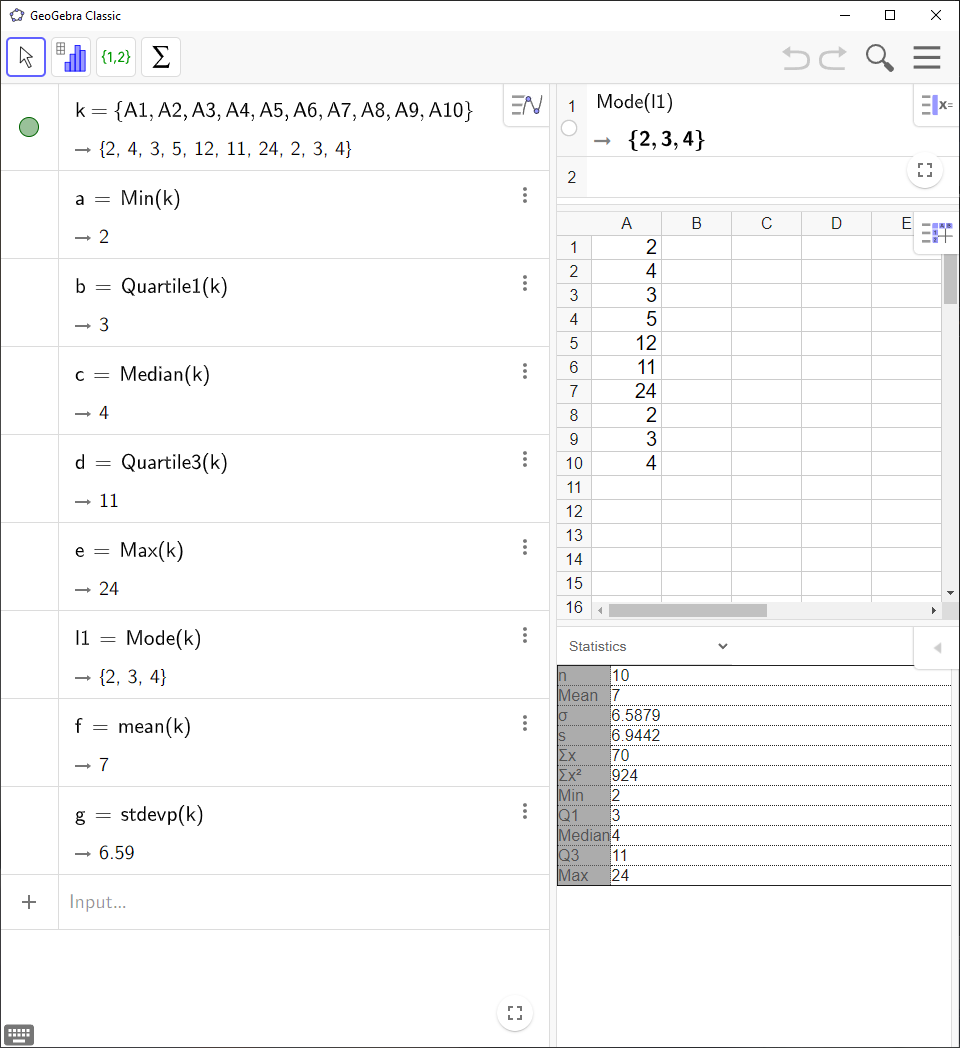# How to Calculate Central Tendencies with GeoGebra

In this entry I’ll show you how you can use `GeoGebra` to find: Minimum, maximum, quartiles, median, mode, mean or standard deviation when you have a list of raw data. You can also find them individually by using commands.

The mode does not show up if you use `One Variable Analysis`. You have to use a command to find it instead.

`GeoGebra` Instruction 1

### Using`One Variable Analysis`1.
Open `Spreadsheet` under`View` in`Menu`.
2.
Enter you data in column `A`.
3.
Select all cells with data in them.
4.
Click `One Variable Analysis`.
5.
Now you get a window with a chart. Click the `Show Statistics`button. If you can’t see the button, click the small triangle in the top right corner of the new window.
6.
Now you get a table showing these statistics:

• $\sigma$ = standard deviation (population)

• `s` = standard deviation (sample),

• `Min` = mininum,

• `Max` = maximum,

• `Q1` = the first quartile,

• `Q3` = the third quartile.

The median and mean are also provided.

`GeoGebra` Instruction 2

### UsingCommands

• Open `Algebra View` and `Spreadsheet` under`View` in`Menu`.

• Enter you observations in column `A` in `Spreadsheet`. Select all your cells and click `List`in `Toolbar`. Change `Name` to `Data` and click `OK`. The list will be saved in `Algebra View`.

• To find the minimum, use the command

Min(<List>)

or

Min(<List of Data>, <List of Frequencies>)

• To find the first quartile, use the command

Quartile1(<List of Raw Data>)

or

Quartile1(<List of Numbers>, <List of Frequencies>)

• To find the median, use the command

Median(<List of Raw Data>)

or

Median(<List of Numbers>, <List of Frequencies>)

• To find the third quartile, use the command

Quartile3(<List of Raw Data>)

or

Quartile3(<List of Numbers>, <List of Frequencies>)

• To find the maximum, use the command

Max(<List>)

or

Max(<List of Data>, <List of Frequencies>)

• To find the mode, use the command

Mode(<List of Numbers>)

• To find the mean, use the command:

mean(<List of Raw Data>)

• To find the variance, use the command

Variance(<List of Numbers>)

• To find the standard deviation, use the command

stdev(<List of Raw Data>)

to get the standard deviation of a sample, or

stdevp(<List of Raw Data>)

to get the standard deviation of a population.